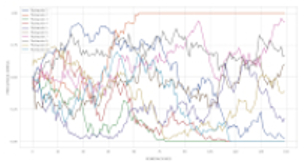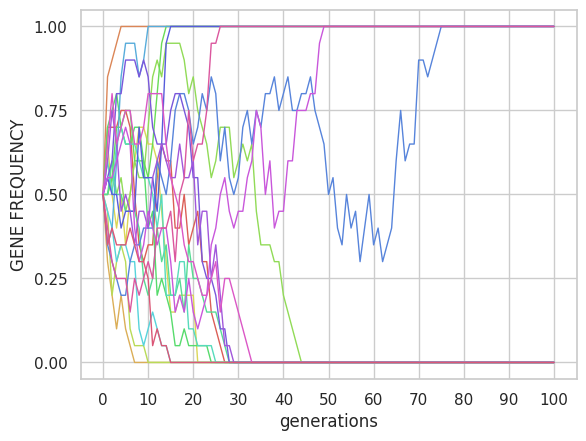﻿ 1LocusSim Manual and Exercises
1LocusSim: SIMULATION OF A LOCUS WITH GENETIC DRIFT, MUTATION AND SELECTIONFeatures
1. 1LocusSim is a simple and adaptable (mobile-friendly) simulator to visualize the effect of genetic drift, selection and mutation on allele frequency.
2. It is programmed in Python based on the NumPy library.

Contact

Back to AC-R home
Simulation of selection
Natural selection
Natural selection under the basic model of a biallelic gene (locus) with a deleterious allele will tend to decrease its frequency. Therefore, the variance σ2 of the allele frequency will decrease. This can be measured by calculating the variance of allele frequency for a set of populations or replicates (Figure 3). The effect is clear if the population size, N, is large (the effective population size Ne, even if it is less than N due to selection, it will remain relatively large). On the contrary, if the population size is small, the deterministic effect of the selection will be overcome by the randomness generated by genetic drift, so that the frequency of the deleterious allele will depend more on chance. For example, with 20 populations and 100 generations we can see in Figure 3 the comparison of the selection effect between the case N = 10 and N=1000 for a coefficient of selection s = 0.1 and dominance h=0.5.Figure 3. Effect of selection on allelic frequency. Parameters: μ=0, s=0.1, h=0.5.

A. Carvajal-Rodriguez - Departamento de Bioquímica Genética e Inmunología - Universidad de Vigo. ( Updated: March 2023)